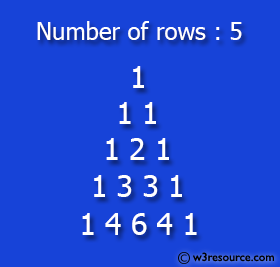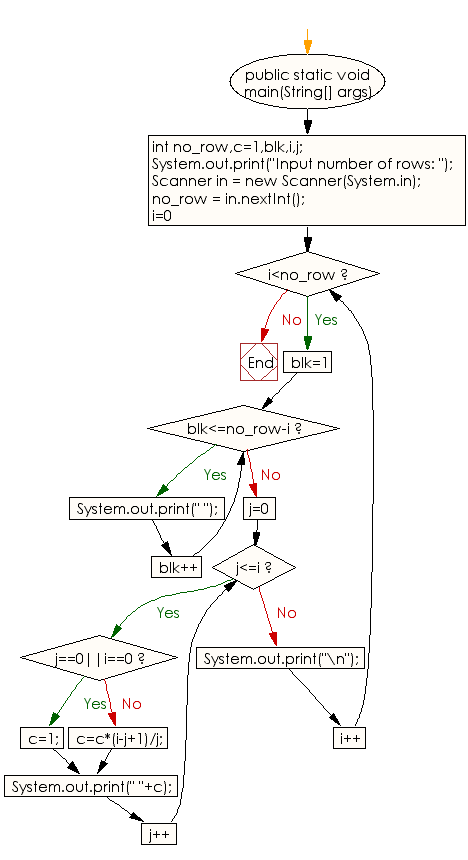﻿ Java exercises: Display Pascal's triangle - w3resource# Java Conditional Statement Exercises: Display Pascal's triangle

## Java Conditional Statement: Exercise-22 with Solution

Write a Java program to display Pascal's triangle.

Test Data
Input number of rows: 5

Pictorial Presentation:Sample Solution:

Java Code:

``````import java.util.Scanner;
public class Exercise22 {

public static void main(String[] args)
{
int no_row,c=1,blk,i,j;
System.out.print("Input number of rows: ");
Scanner in = new Scanner(System.in);
no_row = in.nextInt();
for(i=0;i<no_row;i++)
{
for(blk=1;blk<=no_row-i;blk++)
System.out.print(" ");
for(j=0;j<=i;j++)
{
if (j==0||i==0)
c=1;
else
c=c*(i-j+1)/j;
System.out.print(" "+c);
}
System.out.print("\n");
}
}
}
```
```

Sample Output:

```Input number of rows: 5
1
1 1
1 2 1
1 3 3 1
1 4 6 4 1
```

Flowchart:Java Code Editor:

What is the difficulty level of this exercise?

﻿

## Java: Tips of the Day

How to split a String by space?

You can use the whitespace regex:

```str = "Hello I'm your String";
String[] splited = str.split("\\s+");
```

This will cause any number of consecutive spaces to split your string into tokens.

Ref: https://bit.ly/3xk42If